# Short Primer on Probability

## Fundamentals

A random variable is denoted in capital,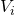and the values it can take is denoted in small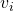.

Consider a collection of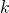random variables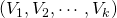. Random variables can be thought of as features of a particular domain of interest.

For example, the result of a coin toss can be represented using a single random variable,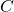. This variable can take either of the categorical values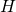or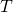. If the same coin is tossedtimes, this can be represented usingvariables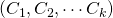. Each of these values can be eitheror.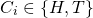## Joint Probability

An expression of the form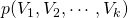is called a joint probability function over the variables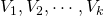. The joint probability is defined when the values of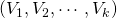are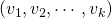respectively. This is denoted by the expression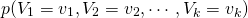This is sometimes abbreviated as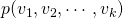.

For a fair coin toss,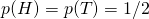. If a fair coin is tossed five times,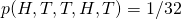.

The joint probability function satisfies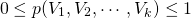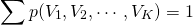## Marginal Probability

The marginal probability of one of the random variables can be computed if the values of all of the joint probabilities for a set of random variables are known.

For example, the marginal probability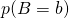is defined to be the sum of all those joint probabilities for which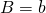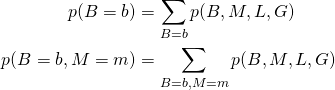When dealing with propositional variables (True/False)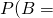True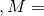Falseis denoted as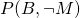.

## Conditional Probabilities

The conditional probability ofgiven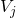is denoted by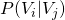.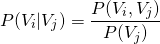where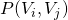is the joint probability ofandand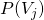is the marginal probability of. Thus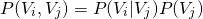Joint conditional probabilities of several variables conditioned on several other variables is expressed as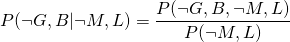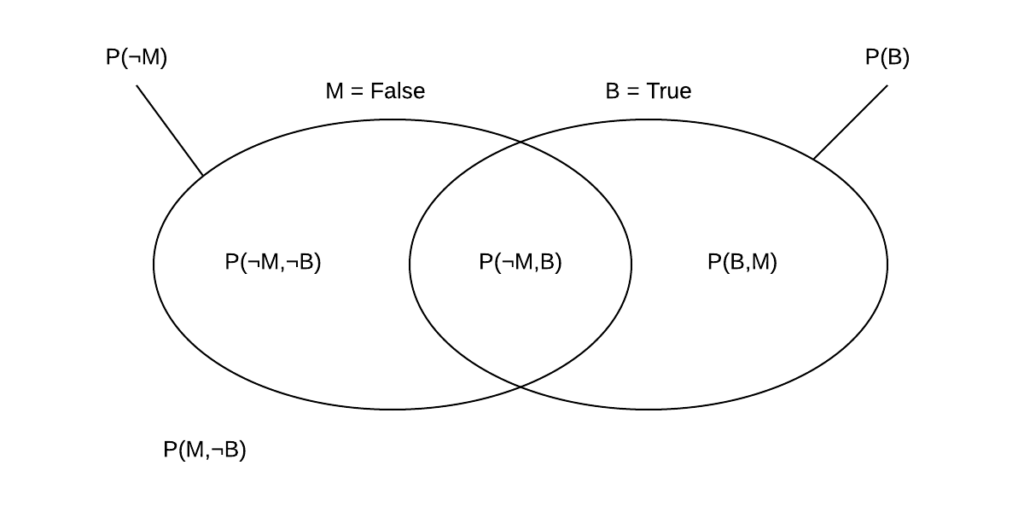Venn diagram clearly illustrating conditional probability. Calculating marginal probability from joint probabilities is obvious. .

A joint probability can be expressed in terms of a chain of conditional probabilities.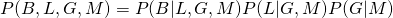The general form of this chain rule is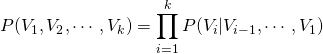## Bayes Rule

Different possible orders give different expressions but they all have the same value for the same set of variable values. Since the order of variables is not important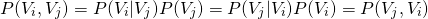Which gives Bayes’ Rule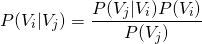## Probabilistic Inference

In set notation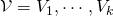,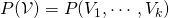, The variables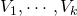having the values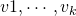respectively is denoted by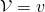, where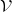and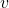are ordered lists.

For a set, the variables in a subset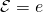ofare given as evidence.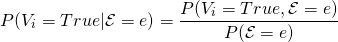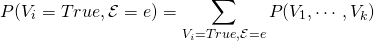For example, consider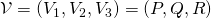. The evidence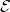is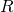being false. In other words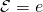equates to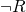.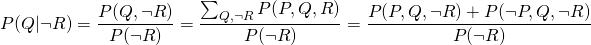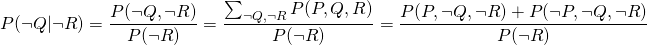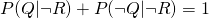Thus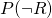need not be computed.

## Conditional Independence

A variable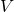is conditionally independent of a set of variables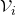given a set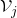if

 (1)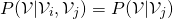tells nothing more aboutthan is already known by knowing.

 (2)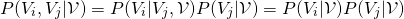Saying thatis conditionally independent ofgivenalso means thatis conditionally independent ofgiven. The same result also applies to setsand.

As a generalization of pairwise independence, the variables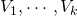are mutually conditionally independent, given a setif each of the variables is conditionally independent of all of the others given.

 (3)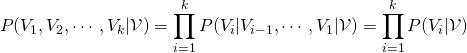Whenis empty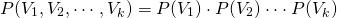This implies that the variables are unconditionally independent.

### Thank You

• Mark – for pointing out typos and errors

## 2 Comments →Short Primer on Probability

1.Mark

Another great article! Nice, clear explanations.

I do have some suggestions for improvement though:

It seems there is an error in the Bayes Rule section. It should be P(V_i|V_j) = P(V_j|V_i)P(V_i)/P(V_j)

I would also recommend explaining how you introduced the P and not P random variables in the Probabilistic Inference section as it is not quite clear how that works out.

Finally, it seems your Latex implementation had trouble rendering for the fourth paragraph in the Conditional Independence section (\textbf{mutually conditionally independent}), right above equation (3).

1.TheBeard

I have expanded the Probabilistic Inference section and fixed the errors you have pointed out. Thank you.